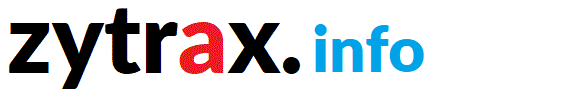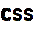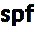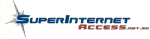tech stuff  |

# Tech Stuff - Acoustic Calculators

These calculators are written in Javascript (saves our bandwidth). Find the calculation you want, and enter the various parameters required then click the 'Calculate' button. If you don't like 'em or think you can improve 'em, the code for the calculators is here. For the equations used in each calculator follow the Notes and Equations Used link.

1. Musical Notes - Table of notes in the range of human hearing to selectable decimal places and with user defined A4 frequency.
2. Center Frequencies - center and band frequencies for octave filters (1 - 1/48).
3. FFT/DFT Parameters - Duration, center and band frequencies for a user defined DFT/FFT size, sample rate and bit-depth.

Serious Health Warning: In all cases the equations used can be found by following the link in the calculator. Standard algorithms are used where they are known and their source documented. Please read the Notes and Equations Used section for these calculators before use.

<OUCH>The calculator did not always display the full range of results between the user supplied upper and lower limits when using anything other than 1000Hz as a base frequency. It now does. Many thanks to Adam Liberman for taking the time to point out this problem.</OUCH>

<OUCH II>The Fractional Octave Centers calculator used the base 2 calculation method defined in IEC 61260/1260 when calculating the edge/crossover frequencies. This can create overlaps and gaps in the crossover frequences at the upper and lower band edges. The base 10 algorithm, also defined in ISO IEC 61260/1260, gives more accurate results and, in general, eliminates the gap/overlap problem. The calculator has been enhanced to provide the base 10 method (while still retaining the base 2 method as an option) and indeed now defaults to use the base 10 method with Calculated centers which is the preferred ISO calculation. Many thanks to Kai Fisher for taking the time to point out this inconsistency.</OUCH>

Musical Notes

Use this calculator to generate the standard western musical notes to a user defined number of places for the 11 Octave range C0 to B10 (Additional Information).

Enter the number of required places in the Decimal Place box (defaults to 2 if no valu supplied). The A4 Pitch is 440 Hz by default (normal International value) but enter any value in the range 430 to 450 Hz if using different tunings. The Number of Harmonics may be any value in the range 2 to 5. Click Calculate when ready. Reset clears all values to their default settings.

The calculator displays the Results as the Note,Fundamental (1st Harmonic), 2nd Harmonic (up to the number requested) in Hertz (a.k.a. Cycles per Second). The comma separted format is ugly but has the overriding merit that it may be copied (or a selected subset), pasted into a text file and imported into a spreadsheet or any other application that supports comma separated format files.

Calculations and equations used.
 Parameters Decimal Places: (range 1 to 5) A4 Pitch: (range 430 to 450) Harmonics: (range 2 to 5) Result

Fractional Octave Centers

Generates the Preferred (Nominal) and Calculated centers (midbands) together with the Upper and Lower band edges (crossover frequencies) for a range of Fractional Octaves and covering a user defined frequency range. These values are typically used in the context of Equalization and filtering.

Depending on the operational parameters being used the results may be ISO'ish. However, as an example, if you change the base frequency from the ISO value of 1000 Hz (default) then the results will clearly not be ISO compatible, even if you are going downhill with a following wind. Use the Calculations and equations used link below for all the gruesome details.

Enter the Decimal Places: for the upper and lower band edge (default is 2). The Calculated center is always shown to 5 decimal places. Select the required Octave Fraction: from the drop down box. Select the required Method: from the drop down list. Calculated Centers - base 10 (ISO) (default) will calculate the Centers (midband), Upper and Lower band edges from the Calculated center using ISO standard Base 10 algorithms. The results in this case (assuming the base frequency remains at the ISO standard of 1000 Hz) will be ISO compatible. Calculated Centers - base 2 (ISO) will calculate calculate the Centers (midband), Upper and Lower band edges from the Calculated center using ISO standard Base 2 algorithms. The results in this case (assuming the base frequency remains at the ISO standard of 1000 Hz) will be ISO compatible. Overlaps or gaps in the adjacent Upper/Lower band edges will result from using this method due to the numerical accuracy of this algorithm. Preferred Centers - base 10 (non-ISO) will calculate Centers (midband), Upper and Lower band edges from the Preferred center using ISO standard Base 10 algorithms. This a non-ISO calculation. Preferred Centers - base 2 (non-ISO) will calculate Centers (midband), Upper and Lower band edges from the Preferred center using ISO standard Base 2 algorithms. This a non-ISO calculation. Calculated Centers - Contiguous (non-ISO) will calculate the Center (midband) using the standard base 2 method but since gaps/overlaps can result it calculates the Upper and Lower band edges using the geometric mean of adjacent band Calculated centers. For each band displayed the Lower band edge is the geometric mean of the Preferred center of the band and the next lowest band and the Upper band is the geometric mean of the Preferred center of the band and the next highest band. This ensures no band gaps or overlaps but is not an ISO approved algorithm. Preferred Centers - Contiguous (non-ISO) provides the same functionality but uses the Preferred center not the Calculated center.

Note: IEC 61260/1260 defines both a base 2 and base 10 calculation methods but the base 10 method is always preferred by the standard due to its greater accuracy. Further, selection of any Method using Preferred frequencies, by virtue of their proximate nature, will yield proximate edge/crossover frequencies! The ISO standard only permits using the Calculated centers.

Set the Lower Limit: and Upper Limit: to any required values - they are defaulted to the audible range (20 Hz and 20000 Hz). The calculator will stop on the Octave boundary after the limits have been exceeded. The Base Frequency default is the ISO value of 1,000 Hz. This may be changed to any non-standard value required but will always be assumed to represent an Octave boundary. Click the Calculate button to see the results. Clicking Reset will set all fields to their default values.

The first line of the Results field displays the parameters used. The second and subsequent lines show a comma separated list of values: Preferred Center frequency, Calculated Center frequency, Lower band limit, Upper band limit (depending on the Method selected the Upper and Lower limits may be computed from the Preferred or Calculated center). The comma separated format is ugly but has the overriding merit that the results may be copied (or a selected subset), pasted into a text editor and imported into a spreadsheet or any other application that supports comma separated format files.

Notes and equations used.
 Parameters Decimal Places: (range 0 to 5) Fractional Octave: 1 Octave Intervals1/3 Octave Intervals1/2 Octave Intervals2/3 Octave Intervals1/6 Octave Intervals1/12 Octave Intervals1/24 Octave Intervals1/48 Octave Intervals Method: Calculated Centers - base 10 (ISO)Calculated Centers - base 2 (ISO)Preferred Centers - base 10 (non-ISO)Preferred Centers - base 2 (non-ISO)Calculated Centers - Contiguous (non-ISO)Preferred Centers - Contiguous (non-ISO) Lower Limit: Hz (range 1 to 100000) Upper Limit: Hz (range 1 to 100000) Base Frequency: Hz (range 10 to 20000) Result

FFT Parameters

Use this calculator to generate the frequency bin width and centers for each output bin (or slot) given a user defined number of input samples to a Discrete Fourier Transform (DFT) or a Fast Fourier Transform (FFT Size) and the sample rate. The Nyquist slot and noise floor (in dB SPL/FS) are also provided.

Enter the number of required places in the Decimal Place box (defaults to 2 if no value supplied). Select the Sample Rate in Hertz (defaults to the CD sample rate or 44100 Hz - 44.1KHz). Select the Bit-Depth (a.k.a Sample Size) (defaults to 16 bits per sample). Select the FFT/DFT Size as the number of samples in the input array of the FFT or DFT (defaults to 2048). Click Calculate when ready. Reset clears all values to their default settings.

The calculator displays the Nyquist Bin (index value starting from 0), the Noise Floor in db SPL/FS (shown as the Dynamic Range based on the sample size + the increase due to the DFT/FFT = Noise Floor), the Bin Width (frequency width of each slot in the FFT ouput array) and the Results showing the bin (or slot) index, frequency value and center frequency for all the slots up to the Nyquist slot. The comma separated format is ugly but has the overriding merit that the results may be copied (or a selected subset), pasted into a text editor and imported into a spreadsheet or any other application that supports comma separated format files.

Notes and equations used. This section also contains a number of formulae that may be useful when dealing with FFT output.
 Parameters Decimal Places: (range 1 to 5) Sample Rate: 8000 Hz (8 KHz)11025 Hz (11.025 KHz)16000 Hz (16 KHz)22025 Hz (22.025 KHz)24000 Hz (24 KHz)32000 Hz (32 KHz)44100 Hz (44.1 KHz)48000 Hz (48 KHz)96000 Hz (96 KHz)192000 Hz (192 KHz) Bit-Depth: 8 bits12 bits16 bits22 bits24 bits32 bits FFT/DFT Size: 128 Samples256 Samples512 Samples1024 Samples2048 Samples4096 Samples16384 Samples32768 Samples65536 Samples Results Bin Width: Hz Nyquist Bin: slot (index from 0) Noise Floor: db (SPL/FS) Bins (or Slots):

# Musical Notes Calculations

1. Each octave is comprised of 12 semi-tones which are displayed as C, C#, D, D#, E, F, F#, G, G#, A, A#, B. Where C# (C sharp) = Db (D flat), D# (D sharp) = Eb (E flat), F# (F sharp) = Gb (G flat), G# (G sharp) = Ab (A flat) , A# (A sharp) = Bb (B flat). We show the # version in all cases for brevity which probably has already sent real musicians into a paroxysm of teeth-gnashing.

2. All notes are assumed to be even tempered (equal intervals) using 1.05946309 as the interval.

3. All notes are derived from an A4 pitch base using a user defined value (defaulted to 440 Hz representing American Standard Pitch). The range C0 to G3# is calculated as lower than the pitch base, A4# to B10 are calculated as higher than the pitch base.

4. Higher notes are calculated as: this Note * 1.05946309 = next highest note

5. Lower notes are calculated as: this Note / 1.05946309 = next lowest note

6. Harmonics are calculated as integer multiples. The 2nd harmonic is 2 x the fundamental (1st harmonic), the 3rd harmonic as 3 x the fundamental etc. to the user defined number (maximum allowed is 5).

# Fractional Octave Calculator

Note: Algorithms and formulae, unless noted otherwise, are taken from IEC 61260/1260:1995, ISO 266:1997 and ISO 3:1973

1. The base frequency (default is ISO 1000 Hz value) is always assumed to be an Octave boundary.

2. When calculating 2/3 Octaves the calculator normalizes all centers to 1/3 from the base frequency and then calculates the edge bands based on the selected set (every 2nd value). This can lead to minor rounding errors.

3. When calculating 1/2 Octaves the calculator normalizes all centers to 1/6 from the base frequency and then calculates the edge bands based on the selected set (every 3rd value). This can lead to minor rounding errors.

4. When using the user selectable Method Calculated Centers - base 10 (ISO) or Preferred Centers - base 10 (non-ISO) Centers are calculated using a base 10 equation:

next lowest center = center / (10 ^ (3 / (10 x interval)))

next highest center = center * (10 ^ (3 / (10 x interval)))

Where interval is 1 for an Octave, 2 for 1/2 Octave, 3 for 1/3 Octave, 6 for 1/6 Octave etc.

5. When using the user selectable Method Calculated Centers - base 2 (ISO), Preferred Centers - base 2 (non-ISO), Calculated Centers - Contiguous (non-ISO) or Preferred Centers - Contiguous (non-ISO) Centers are calculated using a base 2 equation:

next lowest center = center / (2 ^ (1 / interval))

next highest center = center * (2 ^ (1 / interval))

Where interval is 1 for an Octave, 2 for 1/2 Octave, 3 for 1/3 Octave, 6 for 1/6 Octave etc.

6. Centers are always calculated to 5 decimal places. IEC 1260 uses the concept of 5 digit results which may, or may not, include decimal places and thus allows a variable number of decimal places. The calculator will generate more precise values than are required by the standard in all cases.

7. Edge/crossover frequency calculations use either the Preferred center or the Calculated center as defined by the selected Method. The IEC standard mandates use of the Calculated center.

8. The Preferred (Nominal) frequency is taken from the preferred number series R10 for 1, 1/3 and 2/3 Octave values, R20 for 1/6 and 1/2 Octave values, R40 for 1/12 Octave values, R80 for 1/24 and R160 for 1/48 Octave values. Note: The R160 series for 1/48 Octaves does not exist as a standard but is computed by generating a Renard series with an interval of the 160th root of 10 (1.0145) with some manual tweaking which is the same technique apparently (by observation) used by ISO and therefore may be said to be an ISO like Preferred Frequency. The R160 series has a problem in that it can require 3 decimal places to differentiate between adjacent values reliably which may be why ISO has not, in fact, generated such a series. The R160 series uses the R80 values and then 3 decimal places only for those items not covered by R80 and which require the additional resolution.

9. When either Calculated Centers - base 10 (ISO) (default) or Preferred Centers - base 10 (ISO) are selected as the Method the calculator uses the following base 10 ISO formulae to calculate upper and lower edge/crossover frequencies from a single center:

Upper = Center * 10 ^ (3 * number / (10 * 2 x interval))

Lower = Center / 10 ^ (3 * number / (10 * 2 x interval))

Where interval is 1 for an Octave, 3 for 1/3 Octave, 6 for 1/6 Octave etc. and number is the number of required fractions such as 2 for 2/3 Octave, and 1 for 1/6 octaves etc..

10. When either Calculated Centers - base 2 (ISO) or Preferred Centers - base 2 (ISO) are selected as the Method the calculator uses the following base 2 ISO formulae to calculate upper and lower edge/crossover frequencies from a single center:

Upper = Center * 2 ^ (number / (2 x interval))

Lower = Center / 2 ^ (number / (2 x interval))

Where interval is 1 for an Octave, 3 for 1/3 Octave, 6 for 1/6 Octave etc. and number is the number of required fractions such as 2 for 2/3 Octave, and 1 for 1/6 octaves etc..

The above formulas yield two results for each band and a problem due to the algorithm accuracy. The same calculations for adjacent bands never coincide. Thus lower band::high edge != higher band::low edge - there is almost always a gap or an overlap. Due to the nature of analog equalization circuits this problem is probably not terribly significant. In a digital filter this may be much more serious. The calculator provides an alternative, non-ISO, method (labeled Contiguous (non-ISO) in the Method selector) which uses the geometric mean of two adjacent centers as the cutoff frequency using the following equation (assuming band 1 > band 2):

band 1 to band 2 cutoff = Sqrt (band 1 center * band 2 center)

11. While not relevant to the calculator the problem with a single crossover frequency is: which band does it belong to? In the abscence of any other guidance, we use the algorithm:

If target frequency >= this-band::low edge and < this-band::high edge = this-band.

12. The number of octaves in a frequency range f1 to f2 is given by:

no. of octave = (log(f2) - log(f1))/log(2)

13. The center of a band, given the upper and lower bands, is:

Center = (fl x fh)^ 1/2

# FFT/DFT Calculator

This section defines the equations used in calculating the various results and in addition defines a number of formulae that are useful when processing FFT/DFT output.

1. Increase in Noise Floor caused by an DFT/FFT. 10 * log10(FFT size/2).

2. Dynamic Range of an audio sample. 1.72 + (6.02 * number of bits).

3. Frequency Bin Width (frequency covered by each DFT/FFT bin (or slot). Sample Rate in Herz/FFT Size).

4. Frequency for any given bin (or slot). (Bin Index + 1) * Frequency Bin Width.

5. Center Frequency of each bin (or slot). (Bin Index + 1) * Frequency Bin Width + (Frequency Bin Width / 2). Note: The Bin Center is an artificial construct calculated for user convenience. It is not an output from a DFT/FFT.

6. The Nyquist Bin (or slot) is calculated as FFT size / 2 when used as an index value. It is the (FFT Size / 2 + 1)th element in the output array.

7. Normalizing an FFT involves the following calculation on both the real and imaginary parts complex number * 2 / FFT Size. (see explanation).

8. The amplitude of a frequency is calculated as sqrt(real ^ 2 + imaginary ^ 2).

9. The db SPL/FS of a frequency is calculated as 20 * log10(Amplitude). Note: This calculation must be performed on normalized FFT output.

Problems, comments, suggestions, corrections (including broken links) or something to add? Please take the time from a busy life to 'mail us' (at top of screen), the webmaster (below) or info-support at zytrax. You will have a warm inner glow for the rest of the day.

Tech Stuff

If you are happy it's OK - but your browser is giving a less than optimal experience on our site. You could, at no charge, upgrade to a W3C standards compliant browser such as Firefox

 web zytrax.com

#### Our Stuff

SiteCopyright © 1994 - 2023 ZyTrax, Inc. All rights reserved. Legal and Privacy site by zytraxweb-master at zytrax Page modified: January 20 2022.